https://doi.org/10.5194/amt-13-7059-2020
https://doi.org/10.5194/amt-13-7059-2020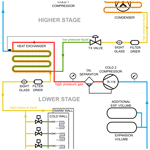# SPIN modification for low-temperature experiments

André Welti, Kimmo Korhonen, Pasi Miettinen, Ana A. Piedehierro, Yrjö Viisanen, Annele Virtanen, and Ari Laaksonen
Abstract

The SPectrometer for Ice Nuclei (SPIN) has been modified to access ice nucleation at low temperatures. The modification consists of a reconfiguration of components from SPIN's cooling system to provide refrigerant with a low boiling point to the chamber. We describe the setup modification and determine the temperature and humidity range accessible to experiments. The modification extends the measurement range of SPIN to 208 K, which enables measurements in the temperature regime relevant for ice formation in cirrus clouds. This addition of low-temperature capability allows for far more comprehensive measurements of the temperature- and humidity-dependent ice nucleation of test substances, to investigate fundamentals of ice nucleation mechanisms. We present exemplary data of heterogeneous ice nucleation on silver iodide and homogeneous ice nucleation in solution droplets to demonstrate the usefulness of the modified SPIN setup for precision measurements to detect discrepancies between experiments and widely used theories.

Share
Dates
1 Introduction

The fundamental understanding of atmospheric ice formation is a complex problem that has been under investigation for almost a century . Part of the complexity of studying ice nucleation experimentally arises from different mechanisms that initiate ice formation at different temperatures and humidities. Tropospheric ice nucleation at low temperatures (T<236K), typical for cirrus clouds, proceeds at water-sub-saturated conditions by homogeneous nucleation of aqueous aerosol or heterogeneous nucleation from the vapour phase. At intermediate temperatures ($\mathrm{236}\phantom{\rule{0.125em}{0ex}}\mathrm{K}) heterogeneous ice nucleation above water saturation leads to glaciation of mixed-phase clouds . Experimental work on heterogeneous ice nucleation mechanisms includes the study of a variety of substances and their ice nucleation potential (e.g. Hoose and Möhler2012), both at water-saturated (immersion freezing, contact freezing) and sub-saturated conditions (deposition ice nucleation, (pore-) condensation freezing). Experimental techniques to study each ice nucleation mechanism have been developed over the years (e.g. DeMott et al.2011). The continuous flow diffusion chamber (CFDC) type of experiment has proven to be a versatile method to isolate and observe different mechanisms, especially ice nucleation from the vapour phase. As pointed out, the advantage of CFDCs is the separate control over temperature and humidity in contrast to expansion or mixing chambers for which T and relative humidity (RH) are interdependent. Often, the dependency of ice nucleation on T and RH by a specific mechanism can be predicted from theoretical considerations (e.g. Fletcher1962; Koop et al.2000; Marcolli2020). Experimental characterization of T- and RH-dependent ice formation is a tool to validate and refine the theories on ice nucleation mechanisms.

We describe a mechanically uncomplicated way to modify the cooling system of the SPectrometer for Ice Nuclei (SPIN) instrument to expand its T and RH range in which experiments can be performed. The modification is beneficial for laboratory studies investigating ice nucleation in a broad T and RH range.

2 SPIN

SPIN is a parallel-plate CFDC manufactured by Droplet Measurement Technologies (DMT). It follows the working principle discussed in with the parallel-plate design from . For a description of the original SPIN instrument and reference experiments, we refer to . Here, the focus is on the compressor cooling system of SPIN, which is the component that was modified for low-temperature operation. The cooling system generates high-pressure, liquid coolant used to cool and maintain the temperature of the chamber wall plates by dosed evaporation of the coolant. In the following, we describe how experimental conditions (T, RH) are generated by controlling the wall plate temperatures and how much the limits of achievable experimental conditions are extended by modifying the cooling system.

## 2.1 Operating principles

Experimental conditions (T, RH) in SPIN are created by coating the parallel (10 mm apart) wall plates of the chamber with a thin (1 mm) ice layer acting as a water reservoir and individually controlling the temperature of the front (warm) and back (cold) plate. The ice-coated walls keep the vapour pressure boundary conditions at ice saturation with respect to the set wall temperature. Under steady-state conditions a linear temperature and water vapour partial pressure gradient establishes between the plates. For an explicit derivation of the linear temperature and water vapour partial pressure field in a CFDC, we refer to and . Because of the non-linear temperature dependence of saturation on water vapour partial pressure (Clausius–Clapeyron), super-saturated conditions can be generated in the 8 mm gap between the ice-covered walls by setting different wall temperatures. For experiments, a lamellar sample, which is confined by a sheath flow to a narrow position between the ice-covered wall plates, is passed through the chamber. T and RH at the lamina position are controlled by the linear temperature and water vapour partial pressure gradient between the cold and warm plate. Figure 1 shows exemplary temperature and water vapour partial pressure gradients in SPIN, at water saturation (RHw=100 %) and T=203, 213, and 223 K at the position of the sample lamina. A ready-to-use code to determine the position of the sample lamina can be found in . For a discussion on sampling bias due to particle displacement outside the lamina, we refer to and .

## 2.2 Modified cooling system

To control the chamber wall temperatures in the original SPIN setup, two independent refrigeration compressor cycles are used. The warm wall is cooled by a single-stage compressor cycle using refrigerant R404A, while the cold wall is cooled by a two-stage (cascade) compressor system with refrigerant R404A in the first, higher stage and refrigerant R116 for the second, lower stage (see Fig. 2 for a cascade compressor diagram). Refrigerant R116 has a boiling point of 194.95 K (at 1 atm pressure) and refrigerant R404A has a boiling point of 226.65 K (at 1 atm pressure). The single-stage compressor cycle using R404A to cool the warm wall plate in the original setup limits the range of T and RH achievable at the sample lamina position. To reach lower temperatures, the SPIN cooling system has been modified by reconnecting the cold wall, cascade compressor system to deliver R116 refrigerant to both wall plates. The configuration of the modified setup is shown in Fig. 2. In practice, three modifications to the cooling system are needed.

• 1.

At the upper part of the chamber, a junction is added to the high-pressure liquid R116 line (lower stage, yellow line in Fig. 2) to connect it to the warm wall plate in parallel to the cold wall.

• 2.

The refrigerant outlet lines of the cold and warm wall, where the refrigerant exits the wall plates in the form of low-pressure gas (lower stage, blue line in Fig. 2), are joined together to return to the cold 2 compressor.

• 3.

Accounting for the increased volume of R116 needed to cool both wall plates, an additional expansion volume (14 L steel tank) is added to the return line (lower stage, blue line in Fig. 2) to give room to the gaseous refrigerant and prevent overpressure when the system is not running.

A consequence of using only one instead of two compressors to deliver the refrigerant for both walls is a reduction in the achievable cooling rate from approximately 2 to 1 K min−1 above 233 K and decreasing to <0.5K min−1 towards the lowest temperatures. Simultaneous cooling of both walls is needed during cooling of the chamber to start an experiment or measurements in which temperature is changed at a constant humidity (T scan). For measurements in which humidity is varied at a constant lamina temperature (RH scan), only one wall plate is cooled, while the other is heated. During such experiments, where most of the refrigerant is used to cool one wall, the cooling rates of the original and modified setups are identical.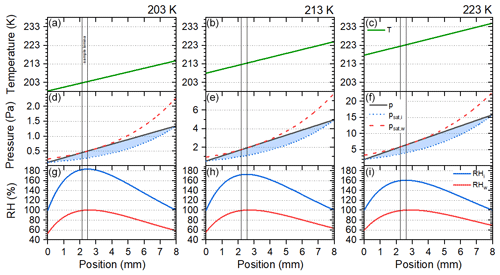Figure 1Linear temperature (a, b, c) and water vapour partial pressure (d, e, f) gradients between the wall plates of SPIN at 203, 213, and 223 K lamina temperature and RHw=100 %. Water vapour partial pressure (p) is compared to the saturation vapour pressure over water (psat, w) and ice (psat, i), calculated according to . Ice super-saturated conditions are indicated as shaded areas. The resulting profiles of relative humidity with respect to ice (RHi) and with respect to water (RHw) are given in the third row (g, h, i). Sample position is indicated by vertical lines.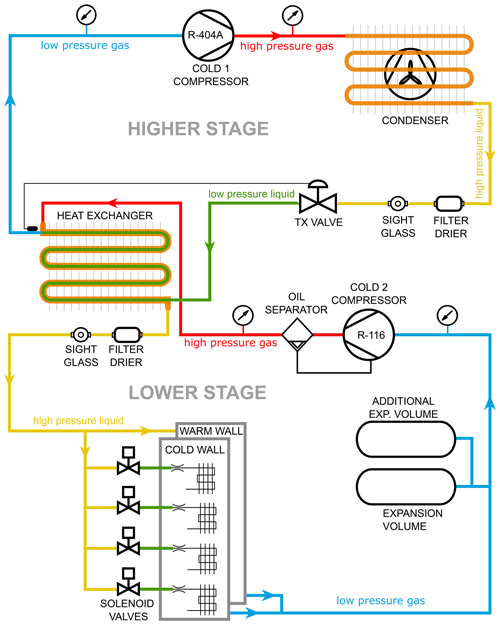Figure 2Diagram of the two-stage cascade compressor setup. Direction of refrigerant circulation is indicated by arrows. Pressure and phase state of the refrigerant are indicated by line colours. For the low-temperature SPIN modification, R116 from the lower stage is used to cool both wall plates, and an additional expansion volume is added.

The ranges of experimental conditions achievable with the original and modified cooling systems of SPIN are shown in Fig. 3. Figure 3a shows the theoretical limits of T and RH conditions, determined according to the examples shown in Fig. 1 by varying the cold and warm wall temperatures from 273.15 to 194.95 K (ice melting temperature to the boiling point of R116). The range of the original SPIN setup is calculated with the cold wall varying from 273.15 to 194.95 K and the warm wall between 273.15 and 226.65 K (boiling point of R404A). Note that ambient heat loss is not considered for the shown range. In practice, the achievable wall temperatures lie 5–10 K above the boiling point of the refrigerant (at 293 K ambient temperature). In addition to instrumental limitations, the conditions under which experiments can be conducted are limited by the optical detection method used to distinguish ice crystals from test aerosol and droplets by size. The SPIN chamber comprises an isothermal section at the end in which conditions are maintained at ice saturation to evaporate droplets . Figure 3a shows the experimentally determined droplet breakthrough conditions where droplets growing above water saturation do not shrink below the size range where particles are classified as ice crystals. Droplet breakthrough limits the explorable humidity range of ice nucleation down to temperatures at which droplets freeze homogeneously. A second limit to detect ice nucleation is caused by the ice crystal growth velocity, which slows down considerably towards lower temperatures due to the lower water vapour partial pressure (cf. second row of Fig. 1) at a certain relative humidity. Conditions for ice crystals to grow to diameters of 0.5, 1, 2 and 4 µm within ∼10 s residence time in the ice super-saturated section of SPIN are calculated according to and shown in Fig. 3b. Details on the calculation of the ice crystal size are included in Appendix A. Ice nucleation experiments often use test aerosol with diameters of up to 1 µm, thus limiting experiments to conditions where the test aerosol can be distinguished from ice crystals to above the 1 µm ice crystal growth conditions shown in Fig. 3b. Note that droplet breakthrough line and ice crystal growth limit the experimental conditions to the same T and RH in any CFDC with dimensions and flows similar to SPIN.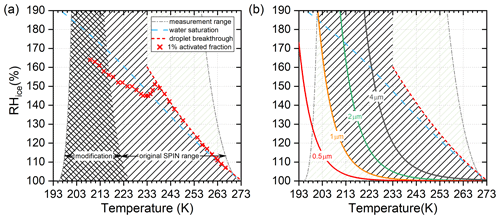Figure 3Calculated range of T and RHi conditions. (a) Extended limit of experimental capacity with the modified SPIN cooling system (cross-hatched area) compared to the original setup (hatched area). Water saturation is indicated as blue dashed line. Red crosses show 1 % activated fractions, measured with 200 nm ammonium sulfate particles (see Fig. 4). Above 235 K the data indicate onset of water breakthrough conditions, below 235 K homogeneous freezing of solution droplets. (b) Limiting conditions to distinguish 0.5, 1, 2 and 4 µm (diameter) test particles from growth-limited ice crystals. Conditions excluded from ice detection by droplet breakthrough and ice crystal growth are lightly hatched. Total experimental range to measure ice nucleation with the modified SPIN setup is shown as a dark hatched area.

3 Laboratory performance

The modified SPIN setup is used to measure the ice nucleation activity of two reference materials in a T and RH range covering all tropospheric ice nucleation conditions. Experiments with ammonium sulfate ((NH4)2SO4) are conducted to measure homogeneous ice nucleation and to determine the droplet breakthrough RH of the setup. Experiments with silver iodide (AgI) are conducted as an example of the setup's utility to investigate heterogeneous ice nucleation.

Aqueous (NH4)2SO4 aerosol is generated with an atomizer (Aerosol Generator Model 3076, TSI) and subsequently dried below the efflorescence point, while AgI particles are dry-generated by agitation of powder with a magnetic stirrer. For both test substances, experiments are performed using dry 200 nm aerosol particles, size-selected with a differential mobility analyser (DMA; TSI Model 3081).

## 3.1 Homogeneous freezing of ammonium sulfate solution

(NH4)2SO4 is a prevalent aerosol throughout the troposphere and commonly used for instrument calibration. Although there are reports of (NH4)2SO4 forming ice heterogeneously at cirrus temperatures , it is often applied as a hygroscopic test substance for forming aqueous aerosol to investigate homogeneous ice nucleation of solutes (e.g. Koop et al.2000).

Figure 4a shows the measured activated fraction (AF) of particles, calculated as the concentration ratio of detected ice crystals exiting SPIN to injected dry particles. Within the limits of detection of the SPIN experiment (approximately $\text{AF}\ge \mathrm{1}×{\mathrm{10}}^{-\mathrm{4}}$), no heterogeneous ice nucleation on solid (NH4)2SO4 particles was observed. The very steep onset of ice formation in dependence on relative humidity below 235 K indicates homogeneous ice nucleation. A comparison of 1 % AF conditions to literature data and the 1 % AF curve derived using the parametrization of is shown in Fig. 4b. Details on the calculation of the homogeneous freezing line are given in Appendix B. While the data are in general agreement with previous observations, there is a clear offset between the slope of the Koop line for 1 % AF and the SPIN data. Homogeneous freezing is observed to set in at lower relative humidities just below 235 K and the temperature-dependent increase in ice super-saturation necessary for constant activity is steeper (almost parallel to the water saturation line). Possible reasons for the discrepancy between the measurements in this study and the Koop parametrization are time-dependent effects; i.e. aqueous aerosol does not reach equilibrium before freezing in SPIN.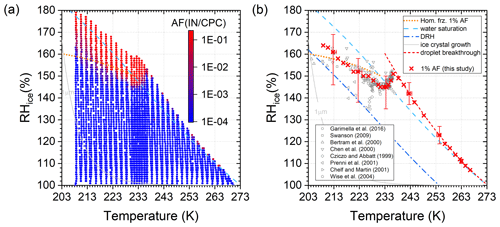Figure 4(a) Activated fraction of 200 nm (NH4)2SO4 particles and (b) 1 % AF in comparison to literature data (references indicated in the figure legend) and the parametrization given in . Lines indicate the Koop line for homogeneous ice formation, water saturation, deliquescence relative humidity (DRH, Seinfeld and Pandis2006), droplet breakthrough and 1 µm ice crystal growth conditions. The range of experimental variability in RHi and T is shown at 10 K intervals.

## 3.2 Heterogenous ice nucleation on silver iodide

Silver iodide (AgI) is an exceptionally active ice-nucleating substance (Vonnegut1947). AgI is widely used for cloud seeding operations to induce glaciation in supercooled liquid clouds and also for seeding of cloud-free, ice super-saturated parts of the atmosphere to generate cirrus clouds . AgI has also served as a model substance to investigate the size and time dependence of ice nucleation and to develop the classical nucleation theory (CNT) of heterogeneous ice formation (Fletcher1959).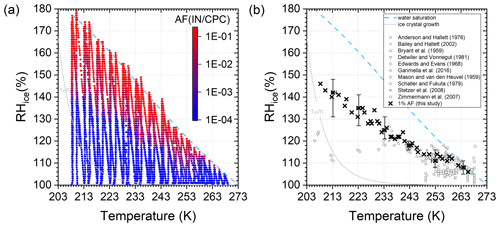Figure 5(a) Activated fraction of 200 nm AgI particles and (b) 1 % AF in comparison to literature data (references indicated in the figure legend). Water saturation and 1 µm ice crystal growth conditions are indicated as lines. The range of experimental variability in RHi and T is shown at 10 K intervals.

Measurements in the entire T–RH space are shown in Fig. 5a. Figure 5b shows a comparison of 1 % AF conditions to literature data. Most previous laboratory experiments were limited to T>233K by the measurement devices. The data collected here show good agreement with the large amount of data in this temperature range. Below 233 K only and previously reported data. The two studies used static diffusion chambers and reported ice nucleation onset conditions without specifying the activated fraction and conditions for 1 % AF, respectively. While the two previous studies observed an inflection in the slope of ice super-saturation needed for a constant amount of ice formation with decreasing temperature, the current study shows a more monotonic increase in ice super-saturation to activate 1 % of the monodisperse AgI particles. A monotonic but less steep increase in ice super-saturation with decreasing temperature for a constant AF is predicted by CNT .

4 Discussion

The modification of the cooling system of SPIN increases the experimentally accessible conditions to the entire T and RH range of ice and mixed-phase cloud formation in the troposphere. Overall, the modification maximizes the measurement range of a CFDC-type instrument with the dimensions of SPIN, since the growth of ice crystals limits the lowest temperature at which ice crystals can be detected and distinguished from dry aerosol (shown in Fig. 3b). In comparison to the original SPIN setup, it allows a broader study of ice nucleation at lower temperature conditions, where ice super-saturation extensively occurs in the atmosphere and ice crystals form before humidities approach water saturation .

To evaluate the performance of the modified SPIN for measuring homogeneous and heterogeneous ice nucleation, experiments using size-selected, 200 nm (NH4)2SO4 and AgI particles are compared to extensive literature data (see Figs. 4 and 5). While the data are generally consistent, the measurements of this study show systematic discrepancies in T and RH dependence both from the predicted heterogeneous ice nucleation of CNT (Fletcher1962) and from the widely used parametrization of homogeneous ice nucleation of solution droplets by . Apart from differences in the method of particle generation, size segregation and detection, it is unclear why partly systematically deviating T and RH dependencies were observed in similar experiments. It highlights the need for comprehensive ice nucleation data sets, measured for a wide range of substances, to improve the understanding of natural and artificial ice nucleation. The automation of the SPIN setup in combination with the extended measurement capability through the modification described here can be a tool to lay the experimental groundwork.

5 Conclusions

We describe a mechanically simple modification of the cooling system of the commercial SPIN instrument. Compared to the cooling system of the original SPIN setup, the modified system has a reduced cooling rate during initial cooling of the chamber in preparation for an experiment; however, cooling rates during experiments are identical. In practice, the modification extends the experimental possibilities by more than 20 K to the full T and RH range of ice nucleation under tropospheric cirrus cloud conditions. This additional temperature range is particularly useful for experiments investigating the temperature and humidity dependence of ice nucleation, since a number of aerosol species only efficiently trigger ice nucleation at such low temperatures. The usefulness of the modified SPIN instrument for laboratory studies is exemplified by characterizing the T and RH dependence of heterogeneous ice nucleation on AgI particles and homogeneous ice nucleation in (NH4)2SO4 solution droplets. From both new data sets a systematic discrepancy between experimentally measured and theoretical predicted ice nucleation is discovered. With the modified SPIN instrument, specific ice nucleation mechanisms can be studied in a broader T and RH range. The instrument is useful for validating theoretical aspects of ice nucleation, and it can be used to investigate ice nucleation on natural and artificial aerosols.

Appendix A: Ice crystal growth

The growth of ice crystals is estimated from the mass growth rate, $\frac{\mathrm{d}m}{\mathrm{d}t}$ :

$\begin{array}{}\text{(A1)}& \frac{\mathrm{d}m}{\mathrm{d}t}=\mathit{\alpha }\cdot \mathrm{4}\cdot \mathit{\pi }\cdot C\cdot \left(\frac{{S}_{\mathrm{i}}-\mathrm{1}}{{F}_{k}+{F}_{d}}\right),\end{array}$

where α is the accommodation coefficient for water molecules , Si the saturation ratio with respect to ice, and C the particle capacitance which incorporates the size and shape of the ice crystal . Assuming a spherical shape of the ice crystal for simplicity, C equals its radius, C=r (see Lamb and Verlinde2011, for C for different shapes). Fk and Fd in the denominator of Eq. (A1) are

$\begin{array}{}\text{(A2)}& & {F}_{k}=\left(\frac{{L}_{\mathrm{s}}}{{R}_{\mathrm{v}}\cdot T}-\mathrm{1}\right)\cdot \frac{{L}_{\mathrm{s}}}{K\cdot T},\text{(A3)}& & {F}_{d}=\frac{{R}_{\mathrm{v}}\cdot T}{{D}_{\mathrm{v}}\cdot {p}_{\mathrm{sat},\phantom{\rule{0.125em}{0ex}}\mathrm{i}}},\end{array}$

where Ls is the latent heat of sublimation , Rv is the individual gas constant for water vapour , T is temperature, K is the thermal conductivity of air , Dv is the water vapour diffusion coefficient in air , and psat, i is the saturation vapour pressure over ice .

Substituting $\frac{\mathrm{d}m}{\mathrm{d}t}$ with ${\mathit{\rho }}_{\mathrm{i}}\cdot \mathrm{4}\mathit{\pi }{r}^{\mathrm{2}}\frac{\mathrm{d}r}{\mathrm{d}t}$ in Eq. (A1) and rearranging leads to the growth equation in terms of the ice crystal radius:

$\begin{array}{}\text{(A4)}& r\frac{\mathrm{d}r}{\mathrm{d}t}=\mathit{\alpha }\cdot \left(\frac{{S}_{\mathrm{i}}-\mathrm{1}}{{\mathit{\rho }}_{\mathrm{i}}\cdot \left({F}_{k}+{F}_{d}\right)}\right),\end{array}$

where r is the ice crystal radius and ρi is the mass density of ice.

Integration yields the time-dependent radius of an ice crystal:

$\begin{array}{}\text{(A5)}& r=\sqrt{{r}_{\mathrm{0}}^{\mathrm{2}}+\mathrm{2}\cdot \mathit{\alpha }\cdot \left(\frac{{S}_{\mathrm{i}}-\mathrm{1}}{{\mathit{\rho }}_{\mathrm{i}}\cdot \left({F}_{k}+{F}_{d}\right)}\right)\cdot t},\end{array}$

where r0 is the seed particle radius and t is time.

Appendix B: Homogeneous freezing of (NH4)2SO4 solution droplets

The fraction of (NH4)2SO4 solution droplets expected to freeze according to the water activity (aw) dependent, homogeneous nucleation rate parametrization by is given by

$\begin{array}{}\text{(B1)}& \mathrm{FF}=\mathrm{1}-\mathrm{exp}\left(-{J}_{\mathrm{hom}}\left({a}_{\mathrm{w}}\right)\cdot {V}_{\mathrm{d}}\cdot t\right),\end{array}$

where FF is the frozen fraction, Jhom(aw) is the nucleation rate, Vd is the volume of the solution drop, and t is time. The volume Vd of (NH4)2SO4 solution droplets can be calculated based on the aw-dependent growth factor (GF) reported in :

$\begin{array}{}\text{(B2)}& \text{GF}=\mathrm{1.49}+\mathrm{2.81}\cdot \left({a}_{\mathrm{w}}{\right)}^{\mathrm{24.6}},\end{array}$

$\begin{array}{}\text{(B3)}& {V}_{\mathrm{d}}=\frac{\mathrm{4}}{\mathrm{3}}\cdot \mathit{\pi }\cdot \left(r\cdot \text{GF}{\right)}^{\mathrm{3}},\end{array}$

with r the radius of the dry (NH4)2SO4 particle.

The parametrization of Jhom(aw) is reproduced from Table 1 in :

$\begin{array}{}\text{(B4)}& \begin{array}{rl}{\mathrm{log}}_{\mathrm{10}}\left({J}_{\mathrm{hom}}\right)=& \phantom{\rule{0.25em}{0ex}}-\mathrm{906.7}+\mathrm{8502}\cdot \mathrm{\Delta }{a}_{\mathrm{w}}-\mathrm{26}\phantom{\rule{0.125em}{0ex}}\mathrm{924}\cdot \left(\mathrm{\Delta }{a}_{\mathrm{w}}{\right)}^{\mathrm{2}}\\ & \phantom{\rule{0.25em}{0ex}}+\mathrm{29}\phantom{\rule{0.125em}{0ex}}\mathrm{180}\cdot \left(\mathrm{\Delta }{a}_{\mathrm{w}}{\right)}^{\mathrm{3}},\end{array}\end{array}$

with

$\begin{array}{}\text{(B5)}& \mathrm{\Delta }{a}_{\mathrm{w}}={a}_{\mathrm{w}}\cdot \mathrm{exp}\left(\frac{\int \left({v}_{\mathrm{w}}-{v}^{\mathrm{i}}\right)\mathrm{d}p}{R\cdot T}\right)-{a}_{\mathrm{w}}^{\mathrm{i}},\end{array}$

where R is the ideal gas constant. The integral can be approximated by

$\begin{array}{}\text{(B6)}& \begin{array}{rl}& \int \left({v}_{\mathrm{w}}-{v}^{\mathrm{i}}\right)\mathrm{d}p\approx {v}_{\mathrm{w}}^{\mathrm{0}}\cdot \left(p-\frac{\mathrm{1}}{\mathrm{2}}\cdot {\mathit{\kappa }}^{\mathrm{0}}\cdot {p}^{\mathrm{2}}-\frac{\mathrm{1}}{\mathrm{6}}\cdot \frac{\partial {\mathit{\kappa }}^{\mathrm{0}}}{\partial p}\cdot {p}^{\mathrm{3}}\right)\\ & \phantom{\rule{0.25em}{0ex}}\phantom{\rule{0.25em}{0ex}}\phantom{\rule{0.25em}{0ex}}-{v}_{\mathrm{i}}^{\mathrm{0}}\cdot \left(p-\frac{\mathrm{1}}{\mathrm{2}}\cdot {\mathit{\kappa }}^{\mathrm{i}}\cdot {p}^{\mathrm{2}}-\frac{\mathrm{1}}{\mathrm{6}}\cdot \frac{\partial {\mathit{\kappa }}^{\mathrm{i}}}{\partial p}\cdot {p}^{\mathrm{3}}\right),\end{array}\end{array}$

with ${v}_{\mathrm{w}}^{\mathrm{0}}=-\mathrm{230.76}-\mathrm{0.1478}\cdot T+\frac{\mathrm{4099.2}}{T}+\mathrm{48.8341}\cdot \mathrm{ln}\left(T\right)$, ${v}_{\mathrm{i}}^{\mathrm{0}}=\mathrm{19.43}-\mathrm{2.2}×{\mathrm{10}}^{-\mathrm{3}}\cdot T+\mathrm{1.08}×{\mathrm{10}}^{-\mathrm{5}}\cdot {T}^{\mathrm{2}}$, κ0=1.6 GPa−1, $\frac{\partial {\mathit{\kappa }}^{\mathrm{0}}}{\partial p}=-\mathrm{8.8}$ GPa−2, κi=0.22 GPa−1, and $\frac{\partial {\mathit{\kappa }}^{\mathrm{i}}}{\partial p}=-\mathrm{0.17}$ GPa−2, where κ0, κi are the isothermal compressibility of water and ice at ambient pressure. ${v}_{\mathrm{w}}^{\mathrm{0}}$ and ${v}_{\mathrm{i}}^{\mathrm{0}}$ are the molar volume of liquid water and hexagonal ice at ambient pressure.

The internal droplet pressure p (GPa) in Eq. (B6) can be calculated using the GF from Eq. (B2) and the surface tension of the solution droplet (σsol):

$\begin{array}{}\text{(B7)}& p=\left(\frac{\mathrm{2}\cdot {\mathit{\sigma }}_{\mathrm{sol}}}{r\cdot \text{GF}}+{p}_{\mathrm{sat},\phantom{\rule{0.125em}{0ex}}\mathrm{w}}\right)×{\mathrm{10}}^{-\mathrm{9}},\end{array}$

where psat, w is the saturation vapour pressure over water . σsol is given in

$\begin{array}{}\text{(B8)}& {\mathit{\sigma }}_{\mathrm{sol}}=\mathrm{0.0761}-\mathrm{1.55}×{\mathrm{10}}^{-\mathrm{4}}\cdot \left(T-\mathrm{273}\right)+\mathrm{2.17}×{\mathrm{10}}^{-\mathrm{3}}\cdot M,\end{array}$

where M is the molarity of (NH4)2SO4 in the droplet which can be determined from the GF.

${a}_{\mathrm{w}}^{\mathrm{i}}$ in Eq. (B5) is given by

$\begin{array}{}\text{(B9)}& \begin{array}{rl}& {a}_{\mathrm{w}}^{\mathrm{i}}=\mathrm{exp}\\ & \phantom{\rule{0.25em}{0ex}}\phantom{\rule{0.25em}{0ex}}\cdot \left(\frac{\mathrm{210}\phantom{\rule{0.125em}{0ex}}\mathrm{368}+\mathrm{131.438}\cdot T-\frac{\mathrm{3.32373}×{\mathrm{10}}^{\mathrm{6}}}{T}-\mathrm{41}\phantom{\rule{0.125em}{0ex}}\mathrm{729.1}\cdot \mathrm{ln}\left(T\right)}{R\cdot T}\right).\end{array}\end{array}$
Data availability

Data sets are available from the authors upon request.

Author contributions

AW, KK and PM modified the chamber. AW conducted the experiments with contributions from KK and AAP. AW prepared the manuscript with contributions from KK, AAP and AL. All the authors commented on the manuscript. YV, AV and AL acquired funding and supervised the project.

Competing interests

The authors declare that they have no conflict of interest.

Acknowledgements

This work was supported by the Academy of Finland, C-Main project (grant no. 309141), and the Centre of Excellence program (grant no. 30733). We thank Angela Buchholz, Scott Ohde and Frank Sagan for helpful discussions. We acknowledge technical assistance from Mikko Hartikainen.

Financial support

This research has been supported by the Academy of Finland, Luonnontieteiden ja Tekniikan Tutkimuksen Toimikunta (grant no. 309141), and the Academy of Finland, Luonnontieteiden ja Tekniikan Tutkimuksen Toimikunta (grant no. 30733).

Review statement

This paper was edited by Pierre Herckes and reviewed by two anonymous referees.

References

Abbatt, J. P. D., Benz, S., Cziczo, D. J., Kanji, Z., Lohmann, U., and Möhler, O.: Solid Ammonium Sulfate Aerosols as Ice Nuclei: A Pathway for Cirrus Cloud Formation, Science, 313, 1770–1773, https://doi.org/10.1126/science.1129726, 2006. a

Bailey, M. and Hallett, J.: Nucleation effects on the habit of vapour grown ice crystals from −18 to −42C, Q. J. Roy. Meteor. Soc., 128, 1461–1483, https://doi.org/10.1002/qj.200212858304, 2002. a

DeMott, P. J., Möhler, O., Stetzer, O., Vali, G., Levin, Z., Petters, M. D., Murakami, M., Leisner, T., Bundke, U., Klein, H., Kanji, Z. A., Cotton, R., Jones, H., Benz, S., Birkmann, M., Rzesanke, D., Saathoff, H., Nicolet, M., Saito, A., Nillius, B., Bingemer, H., Abbatt, J., Ardon, K., Ganor, E., Georgakopoulos, D. G., and Saunders, C.: Resurgence in Ice Nuclei Measurement Research, B. Am. Meteorol. Soc., 92, 1623–1635, https://doi.org/10.1175/2011BAMS3119.1, 2011. a

Detwiler, A. G. and Vonnegut, B.: Humidity Required for Ice Nucleation from the Vapor onto Silver Iodide and Lead Iodide Aerosols over the Temperature Range −6 to −67C, J. Appl. Meteor., 20, 1006–1012, 1981. a, b, c

Findeisen, W.: Colloidal meteorological processes in the formation of precipitation (Die kolloidmeteorologischen Vorgänge bei der Niederschlagsbildung, translated and edited by: Volken, E., Giesche, A. M., and Brönnimann, S., Meteorol. Z., 24, 443–454, https://doi.org/10.1127/metz/2015/0675, 2015), Meteorol. Z., 55, 121–133, 1938. a

Fletcher, N.: The physics of rainclouds, Cambridge University Press, New York, 1962. a, b

Fletcher, N. H.: On Ice-Crystal Production by Aerosol Particles, J. Meteor., 16, 173–180, https://doi.org/10.1175/1520-0469(1959)016<0173:OICPBA>2.0.CO;2, 1959. a

Garimella, S., Kristensen, T. B., Ignatius, K., Welti, A., Voigtländer, J., Kulkarni, G. R., Sagan, F., Kok, G. L., Dorsey, J., Nichman, L., Rothenberg, D. A., Rösch, M., Kirchgäßner, A. C. R., Ladkin, R., Wex, H., Wilson, T. W., Ladino, L. A., Abbatt, J. P. D., Stetzer, O., Lohmann, U., Stratmann, F., and Cziczo, D. J.: The SPectrometer for Ice Nuclei (SPIN): an instrument to investigate ice nucleation, Atmos. Meas. Tech., 9, 2781–2795, https://doi.org/10.5194/amt-9-2781-2016, 2016. a, b

Garimella, S., Rothenberg, D. A., Wolf, M. J., David, R. O., Kanji, Z. A., Wang, C., Rösch, M., and Cziczo, D. J.: Uncertainty in counting ice nucleating particles with continuous flow diffusion chambers, Atmos. Chem. Phys., 17, 10855–10864, https://doi.org/10.5194/acp-17-10855-2017, 2017. a

Hall, W. D. and Pruppacher, H. R.: The Survival of Ice Particles Falling from Cirrus Clouds in Subsaturated Air, J. Atmos. Sci., 33, 1995–2006, https://doi.org/10.1175/1520-0469(1976)033<1995:TSOIPF>2.0.CO;2, 1976. a

Hoose, C. and Möhler, O.: Heterogeneous ice nucleation on atmospheric aerosols: a review of results from laboratory experiments, Atmos. Chem. Phys., 12, 9817–9854, https://doi.org/10.5194/acp-12-9817-2012, 2012. a

Koop, T., Luo, B. P., Tsias, A., and Peter, T.: Water activity as the determinant for homogeneous ice nucleation in aqueous solutions, Nature, 406, 611–614, 2000. a, b, c, d, e, f, g

Korhonen, K., Kristensen, T. B., Falk, J., Lindgren, R., Andersen, C., Carvalho, R. L., Malmborg, V., Eriksson, A., Boman, C., Pagels, J., Svenningsson, B., Komppula, M., Lehtinen, K. E. J., and Virtanen, A.: Ice-nucleating ability of particulate emissions from solid-biomass-fired cookstoves: an experimental study, Atmos. Chem. Phys., 20, 4951–4968, https://doi.org/10.5194/acp-20-4951-2020, 2020. a

Kulkarni, G. and Kok, G.: Mobile Ice Nucleus Spectrometer, Technical Report No. PNNL-21384, Pacific Northwest National Lab. (PNNL), Richland, WA, US, 2012. a

Lamb, D. and Verlinde, J.: Physics and Chemistry of Clouds, Cambridge University Press, https://doi.org/10.1017/CBO9780511976377, 2011. a

Lohmann, U., Lüönd, F., and Mahrt, F.: An introduction to clouds: From the microscale to climate, Cambridge University Press, https://doi.org/10.1017/CBO9781139087513, 2016. a, b

Lüönd, F.: Experimental Study on Immersion Freezing of Size Selected Mineral Dust Particles, PhD thesis, ETH Zurich, https://doi.org/10.3929/ethz-a-005982778, 2009. a

Marcolli, C.: Technical note: Fundamental aspects of ice nucleation via pore condensation and freezing including Laplace pressure and growth into macroscopic ice, Atmos. Chem. Phys., 20, 3209–3230, https://doi.org/10.5194/acp-20-3209-2020, 2020. a

Murphy, D. and Koop, T.: Review of the Vapour Pressures of Ice and Supercooled Water for Atmospheric Applications, Q. J. Roy. Meteor. Soc., 131, 1539–1565, 2005. a, b, c, d

Pruppacher, H. R. and Klett, J. D.: Microphysics of clouds and precipitation, Kluwer, Dordrecht, The Netherlands, 1997. a

Rogers, D. C.: Development of a continuous flow thermal gradient diffusion chamber for ice nucleation studies, Atmos. Res., 22, 149–181, https://doi.org/10.1016/0169-8095(88)90005-1, 1988. a, b, c

Rogers, R. R. and Yau, M. K.: A short course in Cloud Physics, Pergamon, Tarrytown, NY, 1989. a, b, c

Seinfeld, J. and Pandis, S.: Atmospheric Chemistry and Physics: From Air Pollution to Climate Change, John Wiley and Sons, New York, 2nd edn., 2006. a, b

Skrotzki, J., Connolly, P., Schnaiter, M., Saathoff, H., Möhler, O., Wagner, R., Niemand, M., Ebert, V., and Leisner, T.: The accommodation coefficient of water molecules on ice – cirrus cloud studies at the AIDA simulation chamber, Atmos. Chem. Phys., 13, 4451–4466, https://doi.org/10.5194/acp-13-4451-2013, 2013. a

Stetzer, O., Baschek, B., Lüönd, F., and Lohmann, U.: The Zurich Ice Nucleation Chamber (ZINC)-A New Instrument to Investigate Atmospheric Ice Formation, Aerosol Sci. Tech., 42, 64–74, https://doi.org/10.1080/02786820701787944, 2008.  a

Tsilingiris, P.: Thermophysical and transport properties of humid air at temperature range between 0 and 100 C, Energ. Convers. Manage., 49, 1098–1110, https://doi.org/10.1016/j.enconman.2007.09.015, 2008. a

Vonnegut, B.: The Nucleation of Ice Formation by Silver Iodide, J. Appl. Phys., 18, 593–595, https://doi.org/10.1063/1.1697813, 1947. a

Vonnegut, B. and Maynard, K.: Spray-Nozzle Type Silver-Iodide Smoke Generator for Airplane Use, B. Am. Meteorol. Soc., 33, 420–428, https://doi.org/10.1175/1520-0477-33.10.420, 1952. a

Wise, M. E., Surratt, J. D., Curtis, D. B., Shilling, J. E., and Tolbert, M. A.: Hygroscopic growth of ammonium sulfate/dicarboxylicacids, J. Geophys. Res., 108, 4638, https://doi.org/10.1029/2003JD003775, 2003. a